Prev Up Next

# Broken Wing

The argument below was given by Rod Hagglund.

Consider a fixed digit d, and look at the places where it might occur. Call such places adjacent when they lie in the same row, column, or box. Clearly, no two adjacent vertices are both d.

If the resulting graph contains an odd cycle, then there are two adjacent vertices of the cycle that are both different from d. But then these two vertices have a common neighbour outside the cycle that is d (for they lie in a common area - row, column or box - and that area contains a position that is d).

For each edge, call a common neighbour of its endpoints that has d among its possibilities an 'alternative' and write down a list of all alternatives for all edges. The list is nonempty, and a common neighbour of all alternatives cannot itself have value d. Look at an example.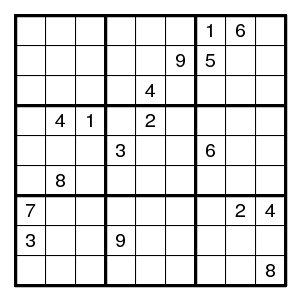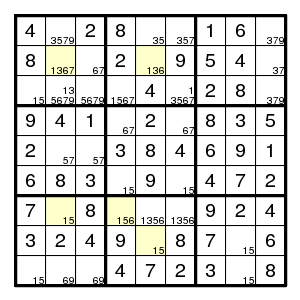The starting configuration leads without problems to the situation on the right. None of the techniques mentioned so far will work here. But there is an odd cycle, here a pentagon, indicated in yellow, of positions that all might contain the same digit 1. By the argument above, there is an edge in the pentagon such that both vertices are different from 1. Now the edge (2,2)-(2,5) must contain at least one 1, since there are no other 1's in that row. Thus an edge where 1's are absent must be one of (2,5)-(8,5), (8,5)-(7,4), (7,4)-(7,2) or (7,2)-(2,2). Alternative choices in the corresponding row, column or box are the (orange) positions (7,5), (7,6) and (3,2), and we know that at least one of them must be 1. But then a common neighbour of each of the alternatives cannot be 1, and in our example (7,2) is such a common neighbour. Therefore (7,2) is not 1.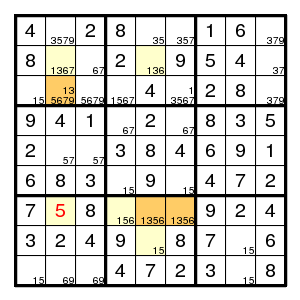The rest is straightforward.

(There are several other ways of describing this situation. For example: if there is a 1 at (7,2) then also at (8,5), and because of the pair (2,2)-(2,5) we find a total of three 1's in columns 2 and 5. Contradiction.)

Usually when Broken Wing applies it is a case where also the simpler Turbot Fish applies. In the above example only one edge of the pentagon is a pair (and Turbot Fish requires at least two such edges).

There is a refinement of the above argument. If an edge of the odd cycle lies in two areas (a row or column and a box), then we need only pick the alternatives from one of these.

Let us give one more example, rather similar to the above one, where also a position outside the pentagon is improved. It is for the digit 4.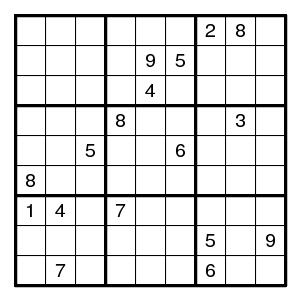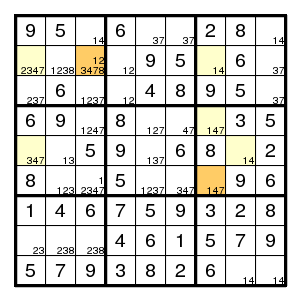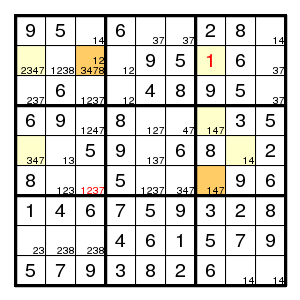The rest is straightforward.

And yet another where only a position outside is improved. Here no edge of the pentagon is a pair. It is for the digit 5.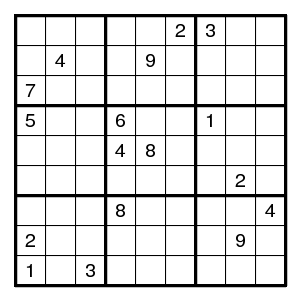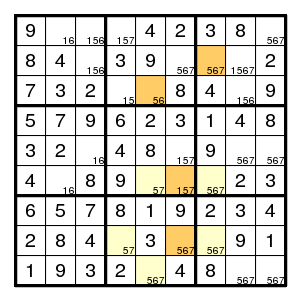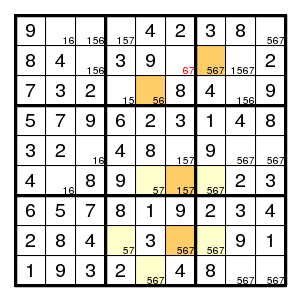Here there remains work to be done. The next step is a forcing chain (5,6)7 > (2,6)6 > (3,5)5 > (6,5)7 > (5,6)!7 where !7 stands for "not 7".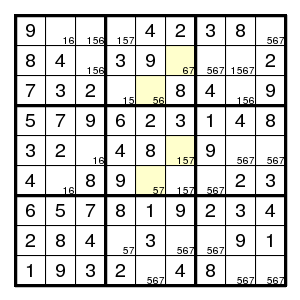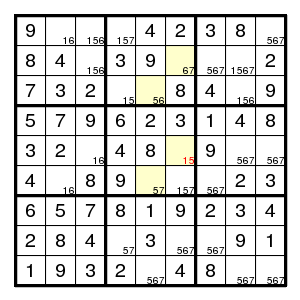The next step is a Turbot Fish for the digit 7.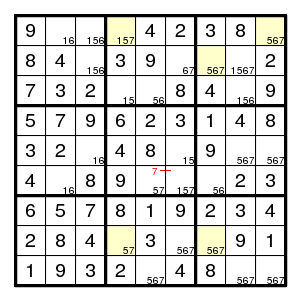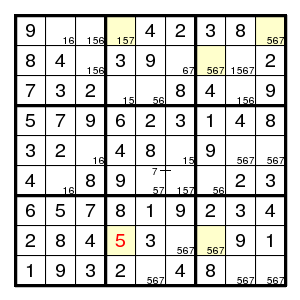The rest is straightforward.